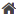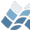NV5 GEOSPATIAL SOFTWARE>  Docs Center  >  Libraries  >  Salvaggio  >  FACET_VERTICES_TO_PLANE_COEFFICIENTS### FACET_VERTICES_TO_PLANE_COEFFICIENTS

FACET_VERTICES_TO_PLANE_COEFFICIENTS

## Name

FACET_VERTICES_TO_PLANE_COEFFICIENTS

## Purpose

This function will compute the coefficients of a plane equation of the form
Ax + By + Cz + D = 0
from a set of triangilar facet vertices. The surface normal can also be
computed if desired.

Graphics.

## Calling Sequence

Result = FACET_VERTICES_TO_PLANE_COEFFICIENTS( vertices,
FACET_NORMAL=facetNormal,
NORMALIZE=normalize )

## Inputs

vertices
A structure containing the vertices of a triangular facet - the tags
are POINT1, POINT2, and POINT3 and these represent the 3-dimensional
coordinates, defined as a 3-element vector, for the vertices in
counter-clockwise order so that the right-hand rule holds for defining
the surface normal

## Keyword Parameters

FACET_NORMAL
This keyword will define a named variable that will contain the surface
normal upon completion of this routine
NORMALIZE
This keyword, used in combination with FACET_NORMAL, will cause the
surface normal vector to be a unit vector

## Result

Result will be a 4-element vector containing the coefficients of the plane
equation of the form [A, B, C, D].

None

NORMALIZE

## Modification History

Written by: Carl Salvaggio
September, 2009 Original code

## Disclaimer

This source code is provided "as is" and without warranties as to performance
or merchantability. The author and/or distributors of this source code may